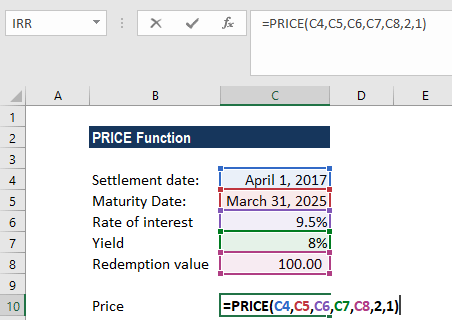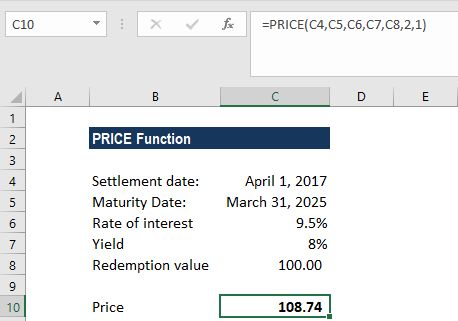# PRICE Function

Calculates the price of a bond per \$100 face value that pays a periodic interest rate

## What is the PRICE Function?

The PRICE Function is categorized under Excel FINANCIAL functions. It will calculate the price of a bond per \$100 face value that pays a periodic interest rate.

In financial analysis, the PRICE function can be useful when we wish to borrow money by selling bonds instead of stocks. If we know the parameters of the bond to be issued, we can calculate the breakeven price of a bond using this function.

### Formula

=PRICE(settlement, maturity, rate, yld, redemption, frequency, [basis])

The PRICE function uses the following arguments:

1. Settlement (required argument) – The bond’s settlement date or the date that the coupon is purchased. The bond’s settlement date should be after the issue date.
2. Maturity (required argument) – This is the bond’s maturity date or the date when the bond expires.

To understand settlement and maturity, let’s take an example: a 30-year bond that was issued on January 1, 2017, and is purchased by a buyer six months later. The issue date would be January 1, 2017, the settlement date would be July 1, 2017, and the maturity date would be January 1, 2047, which is 30 years after the January 1, 2017 issue date.

1. Rate (required argument) – This is the annual coupon rate of the bond.
2. Yld (required argument) – The annual yield of the bond.
3. redemption (required argument) – This is the redemption value of the bond per \$100 face value.
4. Frequency (required argument) – The number of coupon payments per year.
5. Basis (optional argument) – Specifies the financial day count basis that is used by the bond.

BasisDay Count basis
0 or omittedUS(NASD) 30/360
1Actual/actual
2Actual/360
3Actual/365
4European 30/360

### How to use the PRICE Function in Excel?

As a worksheet function, PRICE can be entered as part of a formula in a cell of a worksheet. To understand the uses of the function, let’s consider an example:

#### Example 1

Suppose we wish to calculate the price per \$100 face value of a bond purchased on April 1, 2017. The maturity date of the bond is March 31, 2025. The rate of interest is 9.5%. The yield is 8% and the redemption value is \$100. The US (NASD) 30/360 day count basis is used and payments are made semi-annually. We provide references to the cells in the formula.We get the result below:The above PRICE function returns the value 108.74 (rounded off to 2 decimal points). In the example above:

1. As recommended by Microsoft Excel, we provided as input the settlement and maturity as references to cells containing dates.
2. The interest rate and yield are provided as input in percentage form (9.5% and 8%, respectively). However, the arguments can instead be entered as the simple numerical values 0.095 and 0.08, respectively.
3. If the [basis] argument is omitted, the function will use the default value 0 (denoting the US (NASD) 30/360 day count basis). Here, we provided it as 0.

### A few pointers about the PRICE Function:

1. #NUM! error – Occurs if either:
1. The settlement date is greater than or equal to the maturity date.
2. When we provide invalid numbers for the arguments rate of interest, redemption, frequency, or basis. That is, if the interest rate is less than zero, the yield is less than zero, redemption value is less than or equal to zero, or frequency is any number other than 0,1,2,3,4, or basis is any number other than 0,1,2,3,4.
2. #VALUE! error – Occurs if:
1. The given settlement or maturity arguments are invalid Excel dates.
2. Any of the given arguments are non-numeric.

Thanks for reading CFI’s guide to the Excel PRICE function. By taking the time to learn and master these functions, you’ll significantly speed up your financial analysis. To learn more, check out these additional CFI resources:

• Excel Functions for Finance